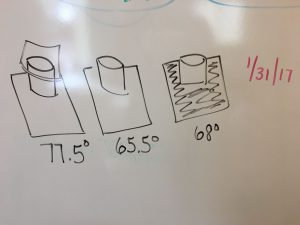# Block 4 – How The World Works

We started with a statement:

Energy can be converted from one form to another allow us to use it in different ways.

We knew we had a LOT of questions right away, so Mrs. Baldwin started writing them all down. But first, we wanted to ask the most important question: WHAT IS ENERGY? We started with what we thought energy might be:

• human energy
• electricity
• fuel
• oil
• fire
• solar (sun)
• hydro (water)
• wind
• food

From some early research, we heard:

• sound is energy
• heat is energy
• there is chemical energy
• gravity
• ALL ENERGY IS POTENTIAL OR KINETIC.

We wanted to do some experiments to help us understand potential and kinetic energy.

Mrs. Baldwin gave us some rubber bands and asked us to stretch them and then freeze. She asked us what would happen if we let go of the stretched rubber bands. We said they would fly across the room! We learned that the rubber band has potential energy when it is stretched, and then kinetic energy when it flies across the room. Kinetic is when something moves.

We did a lot of experiments with potential and kinetic energy. You can read more here: https://storify.com/michellek107/learning-about-potential-kinetic-energy

We performed solar energy experiments and learned that the sun is heat and light energy.We did so many different energy experiments! Light waves, sound waves, reflected light, refracted light, heat, – and we learned so much about how energy travels and is converted.